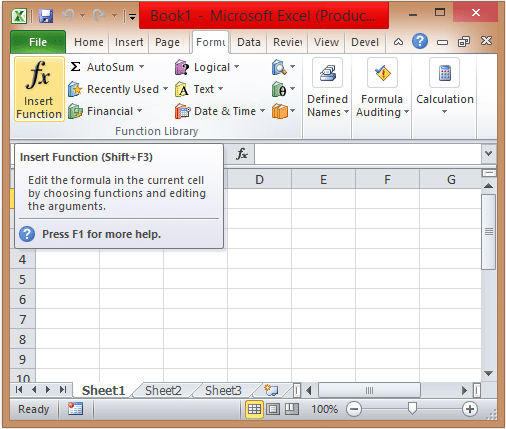To advertise with us contact on Whatsapp: +923041280395 For guest post email at: [email protected]

# Excel Functions List: You Must KnowMicrosoft Excel has many in-built Excel functions list that let you perform calculations and return information about the spreadsheet. These in-built functions are used for performing complex calculations. Here you can see in-built functions for MS Excel that you can use for modifying text, date and time, statistic, and for logical purpose. To get the Excel Functions List as per category, you need to choose the formulas tab and then insert function.

## Excel Functions List

### Statistic functions

AVERAGE: The Average function will return the average of the supplied numbers.

COUNT: It returns the numerical values by counting the value mentioned in the cell.

MIN: It will return the smallest value from the list of the numbers.

MAX: It returns the largest number among the list of the numbers.

SUM: It returns the sum value of all the numbers listed.

COUNTIF: It returns the number of cells which satisfies the given criteria.

### Logical functions

AND: This function will examine the numbers and if all the conditions evaluate to true.

OR: Examine number and returns true if any of the condition shows you true or false.

NOT: It returns the opposite value or expression that is it returns false if the argument is true whereas it returns true if the argument is false.

### Text Functions

TRIM: It removes the duplicate spaces appear at the start and end of the text.

LEFT: It returns the count of characters by running it from start of the text.

RIGHT: It returns the total number of characters of the text starting from the end.

LEN: It returns the total length of the text.

LOWER: It converts all the characters in lower case.

UPPER: It converts all the characters in upper case.

CONCATENATE: This function bind two strings together.

MID: It returns the numbers of character appeared in the middle of the text.

FIND:  It will show you the position of the specified character.

### Date and Time functions

DATE: The date function will return date in year, month, and day format.

TIME: This function will return the time in hour, minute, and second format.

DATEVALUE: The function converts the text of the date into an integer.

TIMEVALUE: It converts the timing text into decimal representing the time.

TODAY: The function will return the current date.

NOW: This function displays the current date and time.

### Financial Functions

CUMIPMT: It returns the outcome of cumulative interest paid.

CUMPRINC: It returns the outcome of cumulative principal paid.

FV: This is the future value of an investment.

NPER: It returns the number of periods for any investment.

### Math & Trig

SQRT: It returns the square root of any given number.

ABS: It returns the absolute value of the number.

MOD: It returns the remainder left after the division of any two numbers.

SIGN: It returns the sign of the number.

### Engineering functions

CONVERT: The function converts a number from one form to another form.

DELTA: This will check whether the two values are equal or not.

ERF: This will return the error function occurred between two limits.

Apart from these Excel functions list, there are other advanced functions available in Excel i.e, conditional formatting excel formula and other advanced functions in excel.